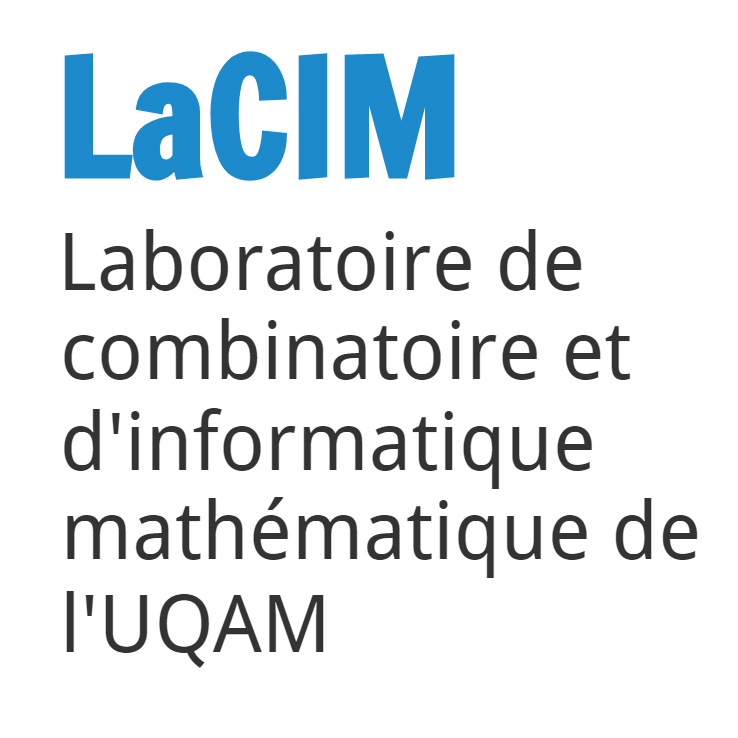# Séminaire du LaCIM: «Quantum groups, quantum Weyl algebras, and a new First Fundamental Theorem of invariant theory for \$U_q(gl(n))\$ »

The First Fundamental Theorem (FFT) is one of the highlights of invariant theory of reductive groups and goes back to the works of Schur, Weyl, Brauer, etc. For the group \$GL(V)\$, the FFT describes generators for polynomial invariants on direct sums of several copies of the standard module \$V\$ and its dual \$V*\$. Then R. Howe pointed out that the FFT is closely related to a double centralizer statement inside a Weyl algebra (a.k.a. the algebra of polynomial-coefficient differential operators).

In this talk we first construct a quantum Weyl algebra and then present a quantum analogue of the FFT. As a special case of this FFT we obtain a double centralizer statement inside our quantum Weyl algebra. We remark that the FFT that we obtain is different from the one proved by G. Lehrer, H. Zhang, and R.B. Zhang for U_q(gl(n)). Time permitting, I will explain the connection between this work and the Capelli Eigenvalue Problem for classical quantum groups. This talk is based on a joint project with Gail Letzter and Siddhartha Sahi.# clockCreated with Sketch.Date / heure

vendredi 2 décembre 2022
11 h à 12 h

# pinCreated with Sketch.Lieu

UQAM - Pavillon Président-Kennedy (PK)
PK-4323
201, avenue du Président-Kennedy
Montréal (QC)